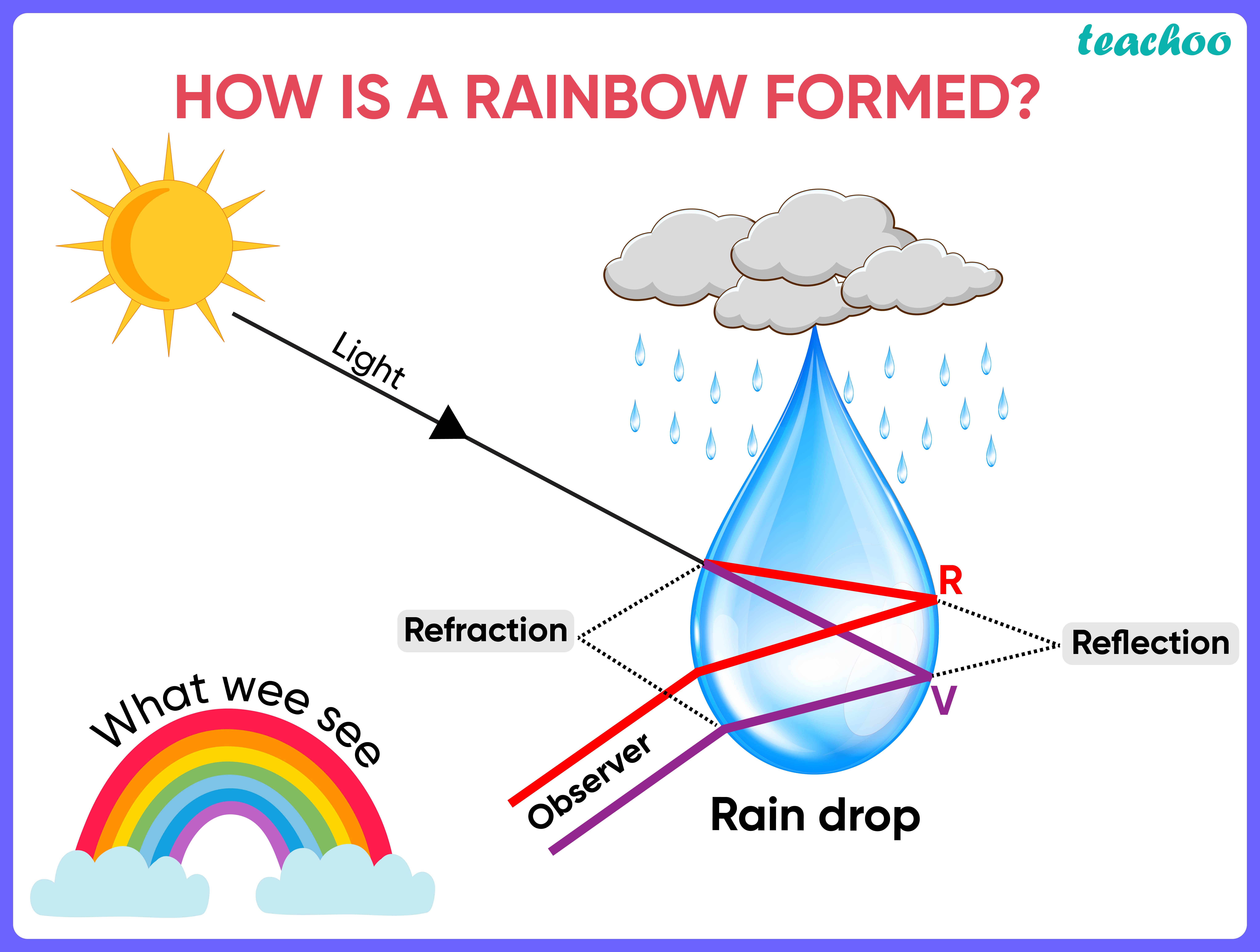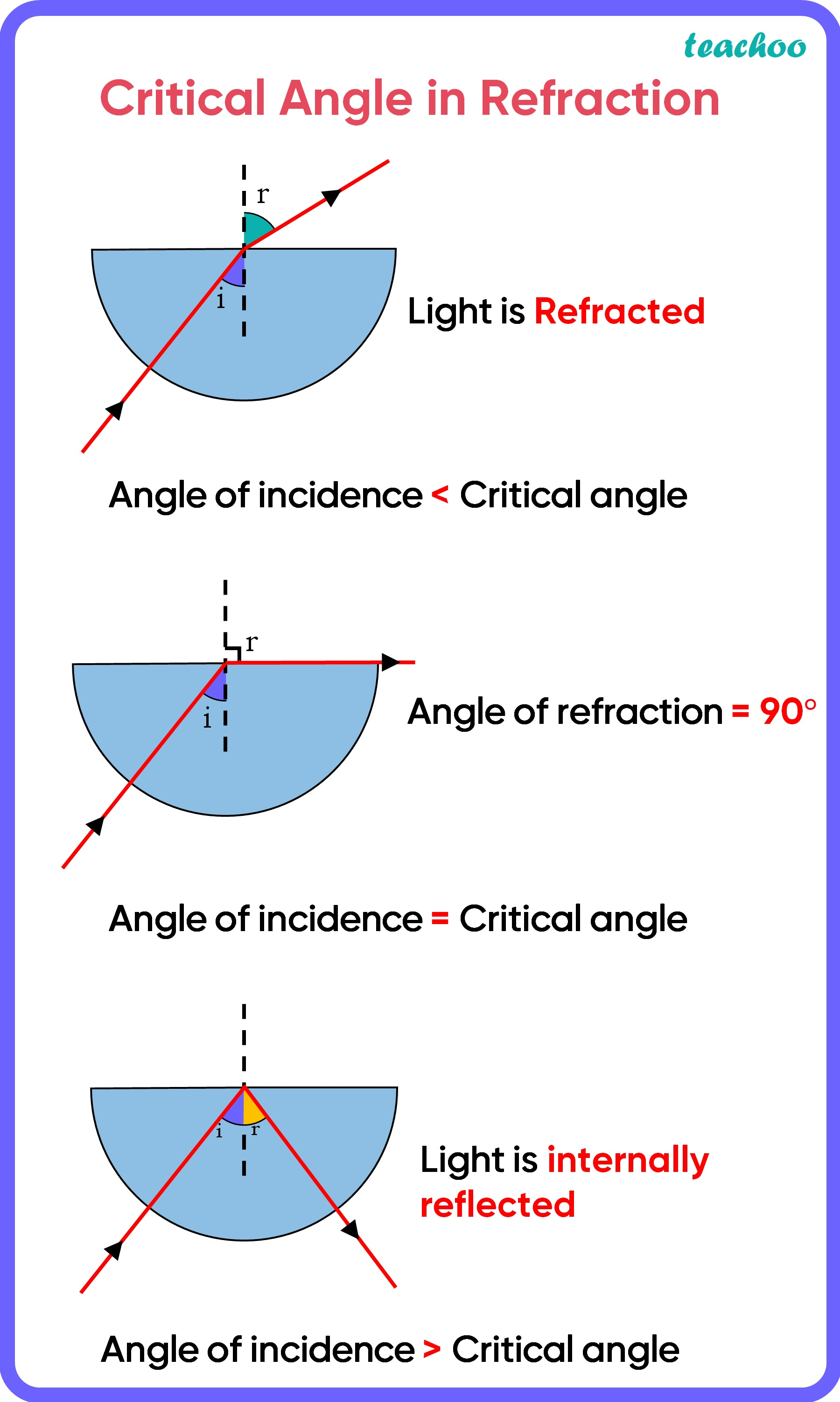Assertion Reasoning Questions (MCQ)

Class 10
Chapter 11 Class 10 - Human Eye and Colourful World

## Reason (R): The angle between the refracted ray  and normal to the drop surface is greater than the  critical angle.

Let’s look at how a rainbow is formed

• After rain , there are many tiny droplets of water still in the air
• When sunlight falls on these droplets
• These droplets act as tiny prisms
• When sun rays fall on these droplets, the rays get first refracted , then internally reflected and then refracted again
• Due to this different colors of sunlight are bent at different angles and we are able to see a spectrum in the form of a rainbowSince light at the inner surface gets internally reflected, Assertion is true

For critical angle,

• Total internal reflection only takes place if the light from a denser medium to a lighter medium strikes at an angle of incidence larger than the critical angle
• If the angle between the refracted light and the normal to the drop surface is greater than a critical angle ( which is 45 degrees), the light reflects off the back of the drop .
• If the angle between the refracted light and the normal to the drop surface is less than the critical angle , the light will simply pass through .Therefore, r easoning is also a true and correct explanation of assertion .

Thus,

• Assertion is True
• Reason is True
• And reason is the correct explanation for the assertion.

So, the correct answer is (a)

Learn in your speed, with individual attention - Teachoo Maths 1-on-1 Class